Call for Papers for the 25th Jubilee Edition of the International Conference on Intuitionistic Fuzzy Sets is now open!
Conference: 9–10 September 2022 • Deadline for submission: 30 May 2022.

Local operators over generalized nets

• temporal operators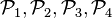$\mathcal{P}_1, \mathcal{P}_2, \mathcal{P}_3, \mathcal{P}_4$ that change temporal components of a given transition,
• matrix operators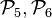$\mathcal{P}_5, \mathcal{P}_6$ that change some ofthe index matrices of a given transition,
•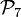$\mathcal{P}_7$ alters the transition's type,
•$\mathcal{P}_8$ alters the capacity of some of the places in the net,
•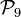$\mathcal{P}_9$ alters the characteristic function of an output place,
•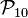$\mathcal{P}_{10}$ alters the evaluation function associated with the transition condition predicates of the given transition.